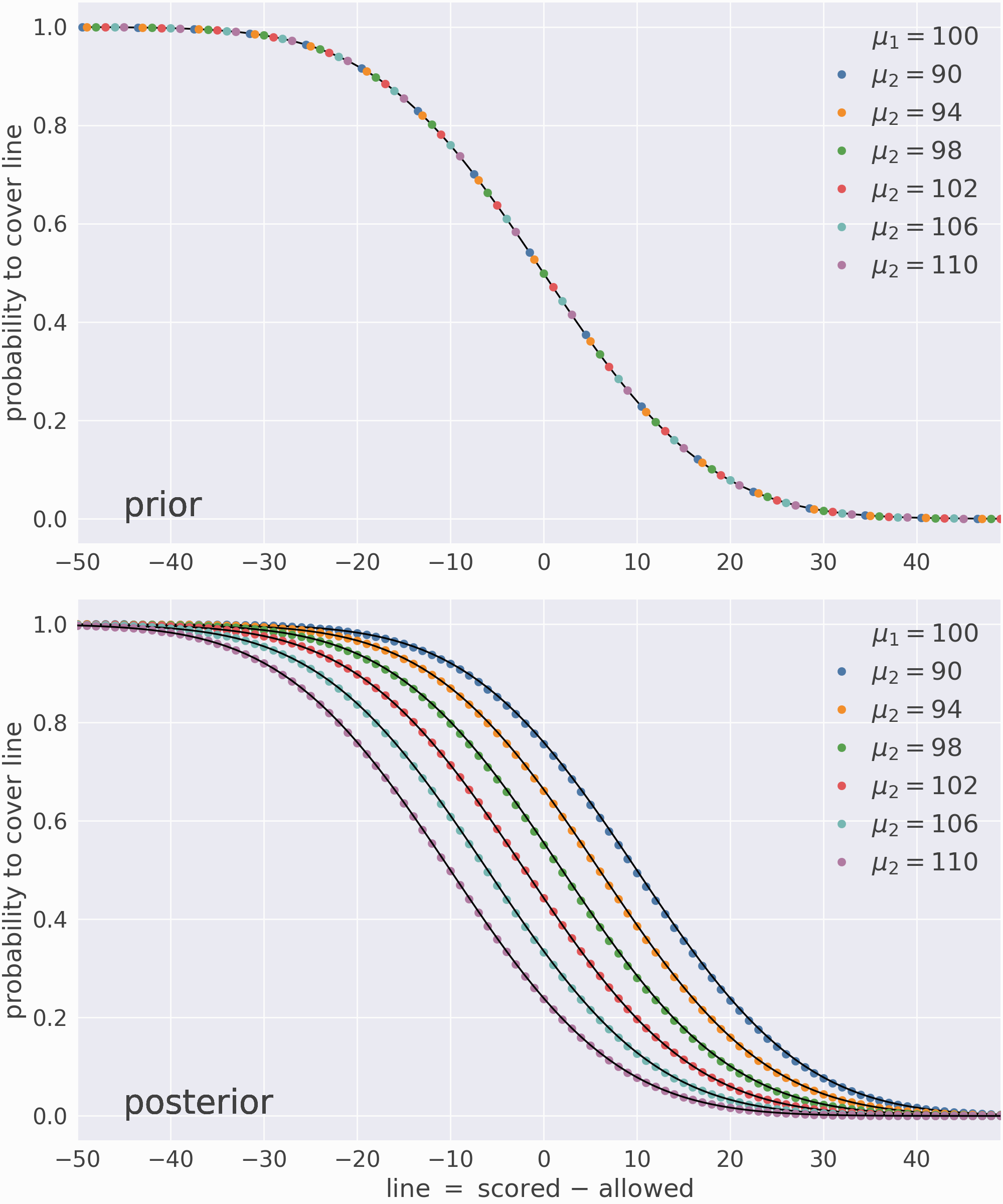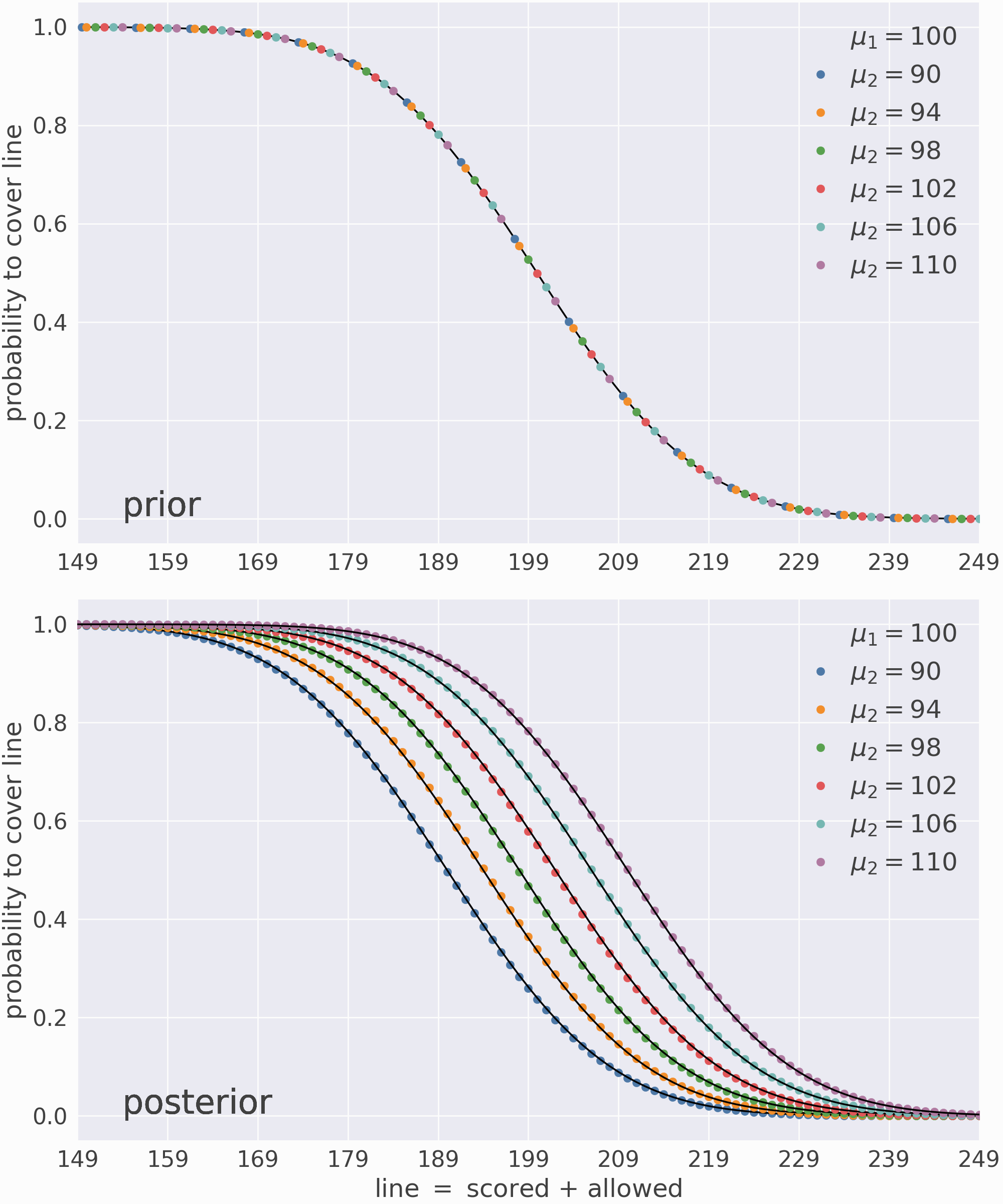# Tests¶

elora has a standard set of unit tests, whose current CI status is:The unit tests do not check the physical accuracy of the model which is difficult to verify automatically. Rather, this page shows a number of statistical tests which can be used to assess the model manually.

## Toy model¶

Consider a fictitious “sports league” of seven teams. Each team samples points from a normal distribution $$X_\text{team} \sim \mathcal{N}(\mu, \sigma^2)$$ where $$\sigma=10$$ is a fixed standard deviation and $$\mu_\text{team}$$ is one of seven numbers

$\mu_\text{team} \in \{90, 94, 98, 100, 102, 106, 110\}$

specifying the team’s mean expected score. I simulate a series of games between the teams by sampling pairs $$(\mu_{\text{team}_1}, \mu_{\text{team}_2})$$ with replacement from the above values. Then, for each game and team, I sample a normal random variable $$\mathcal{N}(\mu_\text{team}, \sigma^2)$$ and record the result, producing a tuple

$(\text{time}, \text{team}_1, \text{score}_1, \text{team}_2, \text{score}_2),$

where time is a np.datetime64 object recording the time of the comparison, $$\text{team}_1$$ and $$\text{team}_2$$ are strings labeling each team by their $$\mu$$-values, and $$\text{score}_1$$ and $$\text{score}_2$$ are random floats. This process is repeated $$\mathcal{O}(10^6)$$ times to simulate a large number of games played between the teams.

I then calculate the score difference or $$\text{spread} \equiv \text{score}_1 - \text{score}_2$$ for each game to form a list of comparisons $$(\text{time}, \text{team}_1, \text{team}_2, \text{spread})$$ and use these comparisons to train the Elo regressor algorithm:

scale = np.sqrt(2*10**2) # std dev for difference of two normal random variables
model = Elora(1e-4, scale=scale, commutes=False)

Now that the model is trained, I can predict the probability that various matchups cover each value of the line, i.e. $$P(\text{spread} > \text{line})$$. Since the underlying distributions are known, I can validate these predictions using their analytic results.